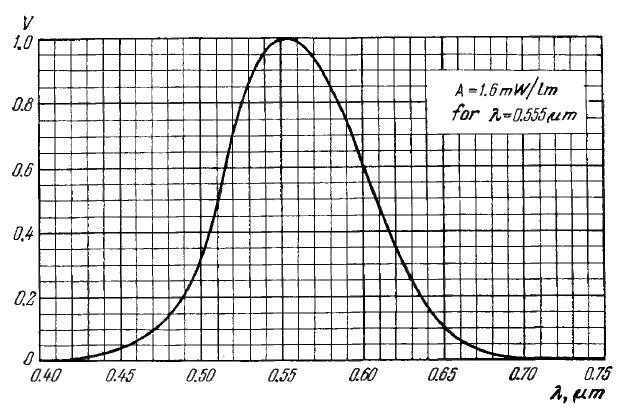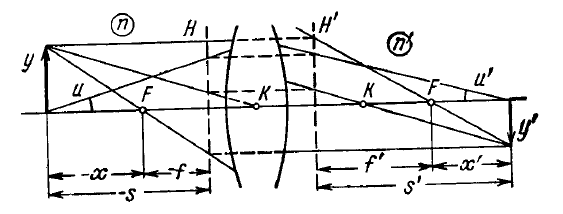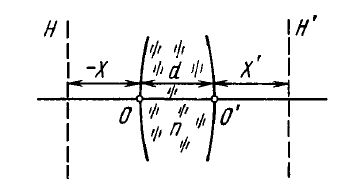# Photometry and Geometrical Optics

For page specific messages
For page author info
• Spectral response of an eye $V(\lambda)$ is shown in Fig. 5.1• Luninous intensity $I$ and illuminance $E$: $$I = \frac{d\Phi}{d\Omega}, E = \frac{d\Phi_{inc}}{dS}.$$
• Illuminance produced by a point isotropic source: $$E=\frac{I\cos(\alpha)}{r^2},$$ where $\alpha$ is the angle between the normal to the surface and the direction to the source.
• Luminosity $M$ and luminance $L$: $$M = \frac{d\Phi_{emit}}{dS}, L=\frac{d\Phi}{d\Omega\.\Delta S \cos(\theta)} • For a Lambert source L = const and luminosity$$M=\pi L.$$• Relation between refractive angle \theta of a prism and least deviation angle \alpha:$$\sin\frac{\alpha+\theta}{2} = n \sin \frac{\theta}{2},$$where n is the refractive index of the prism. • Equation of spherical mirror:$$\frac{1}{s^{\prime}}+\frac{1}{s} = \frac{2}{R},where R is the curvature radius of mirror • Equations for aligned optical system (Fig. 5.2):\frac{n^{\prime}}{s^{\prime}}-\frac{n}{s}=\Phi, \frac{f^{\prime}}{s^{\prime}}+\frac{f}{s} = 1, xx^{\prime} = ff^{\prime}$$• Relations between focal lengths and optical power:$$f^{\prime} = \frac{n^{\prime}}{\Phi}, f=-\frac{n}{\Phi}, \frac{f^{\prime}}{f} = - \frac{n^{\prime}}{n}$$• Optical power of a spherical refractive surface:$$\Phi = \frac{n^{\prime}-n}{R}.$$• Optical power of a thin lens in a refractive index n_0:$$\Phi = (n - n_0)\left(\frac{1}{R_1}-\frac{1}{R_2}\right),$$where n is the refractive index of the lens. • Optical power of a thick lens:$$\Phi = \Phi_1+\Phi_2-\frac{d}{n}\Phi_1\Phi_2,$$where d is the thickness of the lens. This equation is also valid for a system of two thin lenses separated by a medium with refractive index n. • Principal planes h and h^{\prime} are removed from the crest points O and O^{\prime} of surfaces of a thick lens (Fig 5.3) by the following distances:$$X= \frac{d}{n}\frac{\Phi_2}{\Phi}, X^{\prime}= -\frac{d}{n}\frac{\Phi_1}{\Phi}$$• Lagrange-Helmholtz invariant:$$nyu = const.$$• Magnifying power of an optical device:$$\Lambda = \frac{\tan \psi^{\prime}}{\tan\psi}, where $\psi^{\prime}$ and $\psi$ are the angles subtended at the eye by an image formed by the optical device and by the corresponding object at a distance for convenient viewing (in the case of a microscope or magnifying glass is equal to $l_0 = 25cm$).

n
0

X